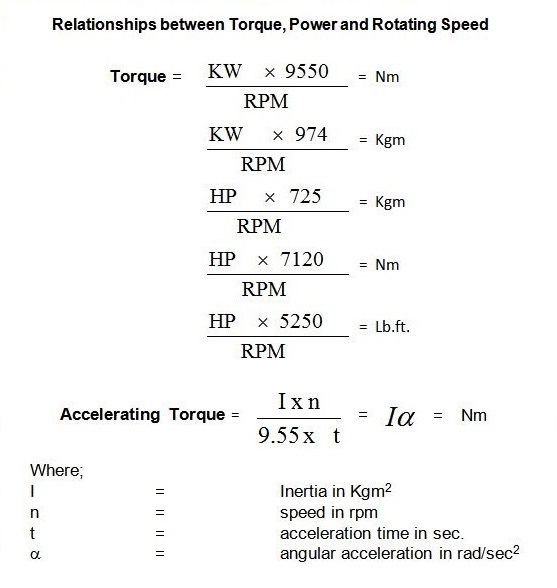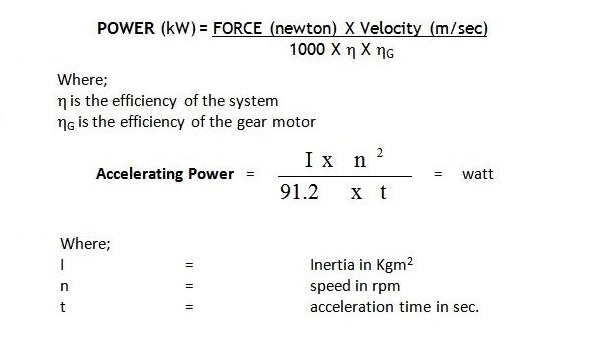# Torque and Power

### Torque

Torque, moment or moment of force, is the tendency of a force to rotate an object about an axis, fulcrum, or pivot. Just as a force is a push or a pull, a torque can be thought of as a twist to an object. Mathematically, torque is defined as the cross product of the lever-arm distance and force, which tends to produce rotation.

#### Torque = Force X Radius### Power

In physics, Power (symbol: P) is defined as the amount of energy consumed per unit time. In the MKS system, the unit of power is the joule per second (J/s), known as the watt (in honor of James Watt, the eighteenth-century developer of the steam engine).

#### Power = Force X Velocity